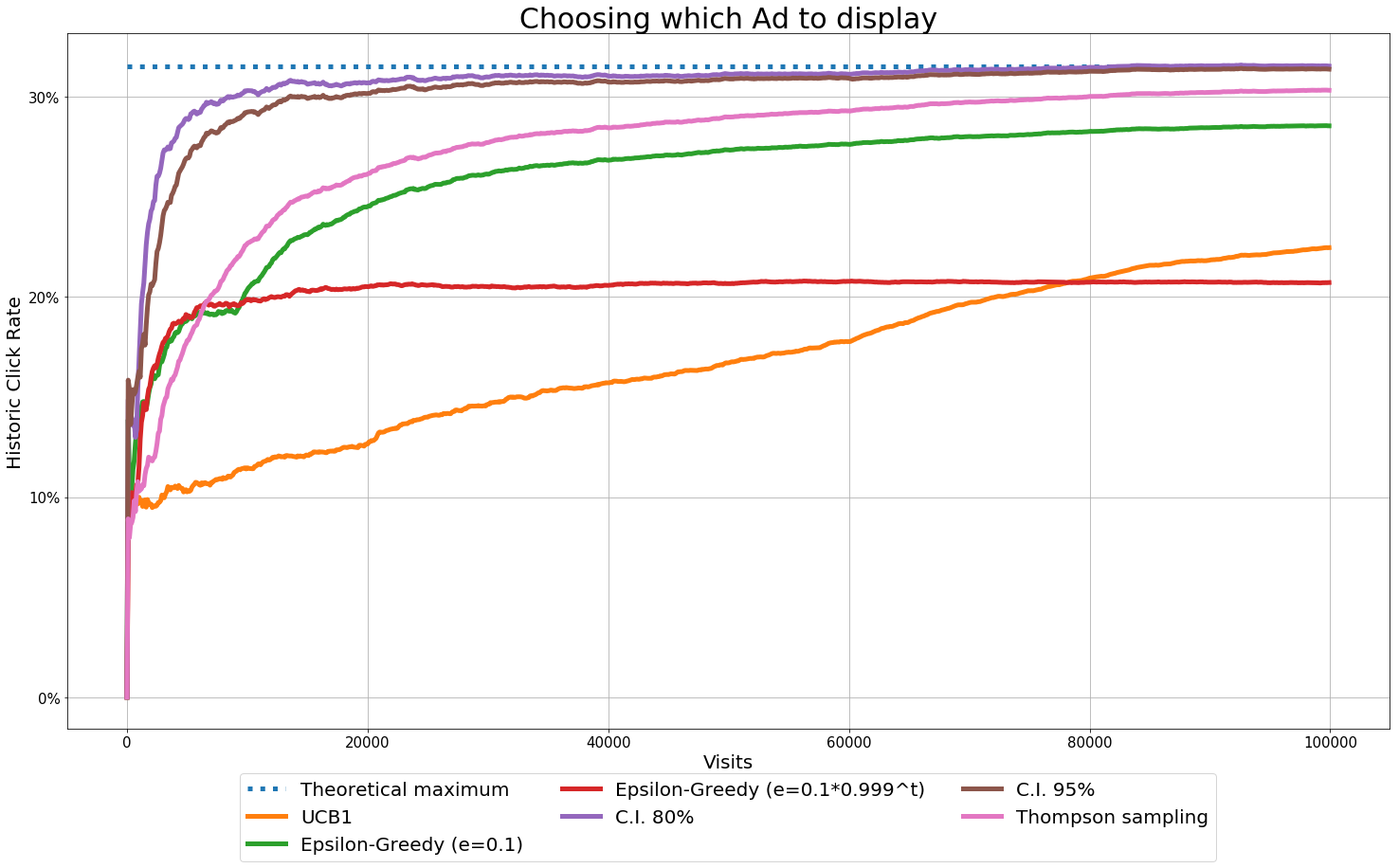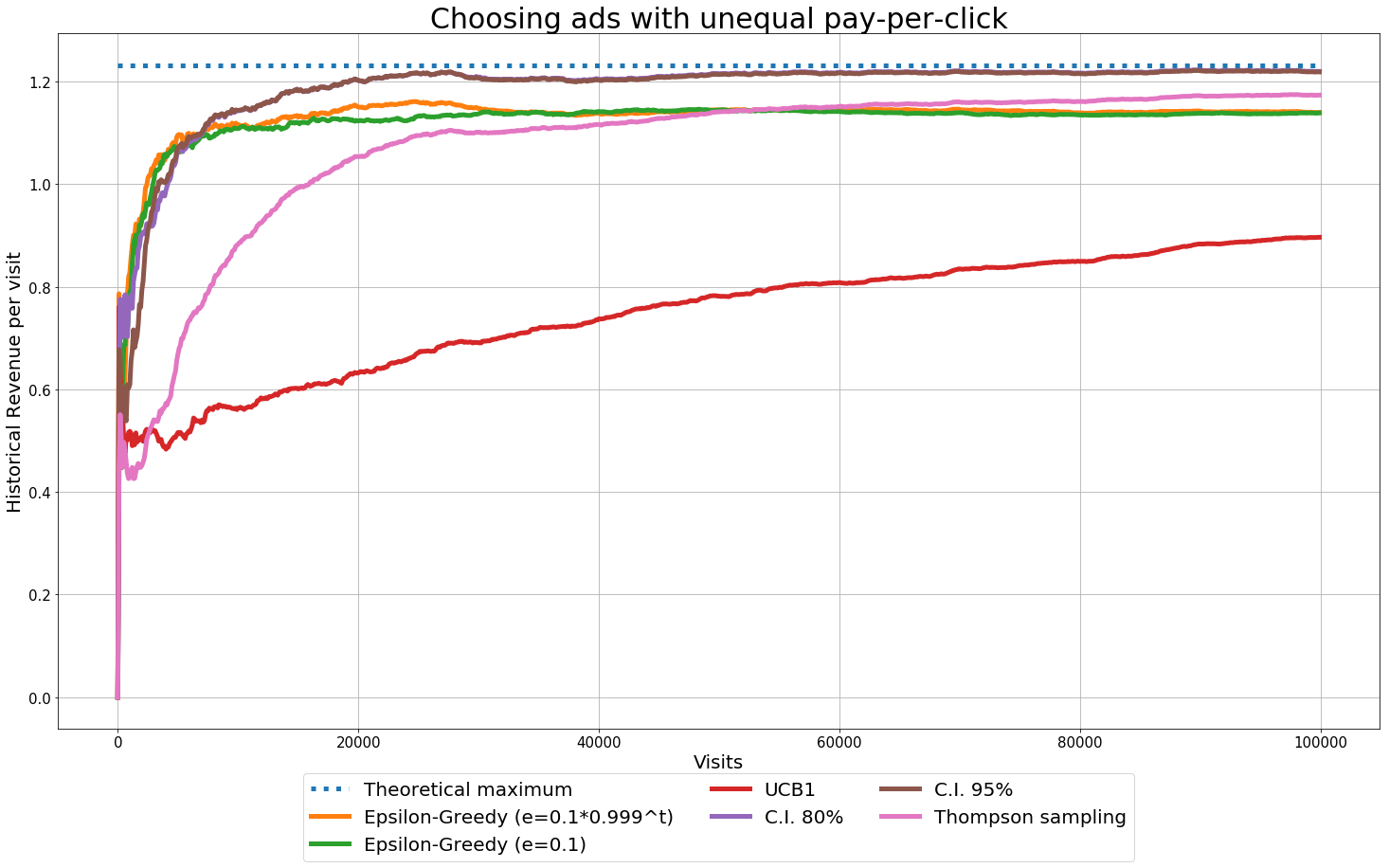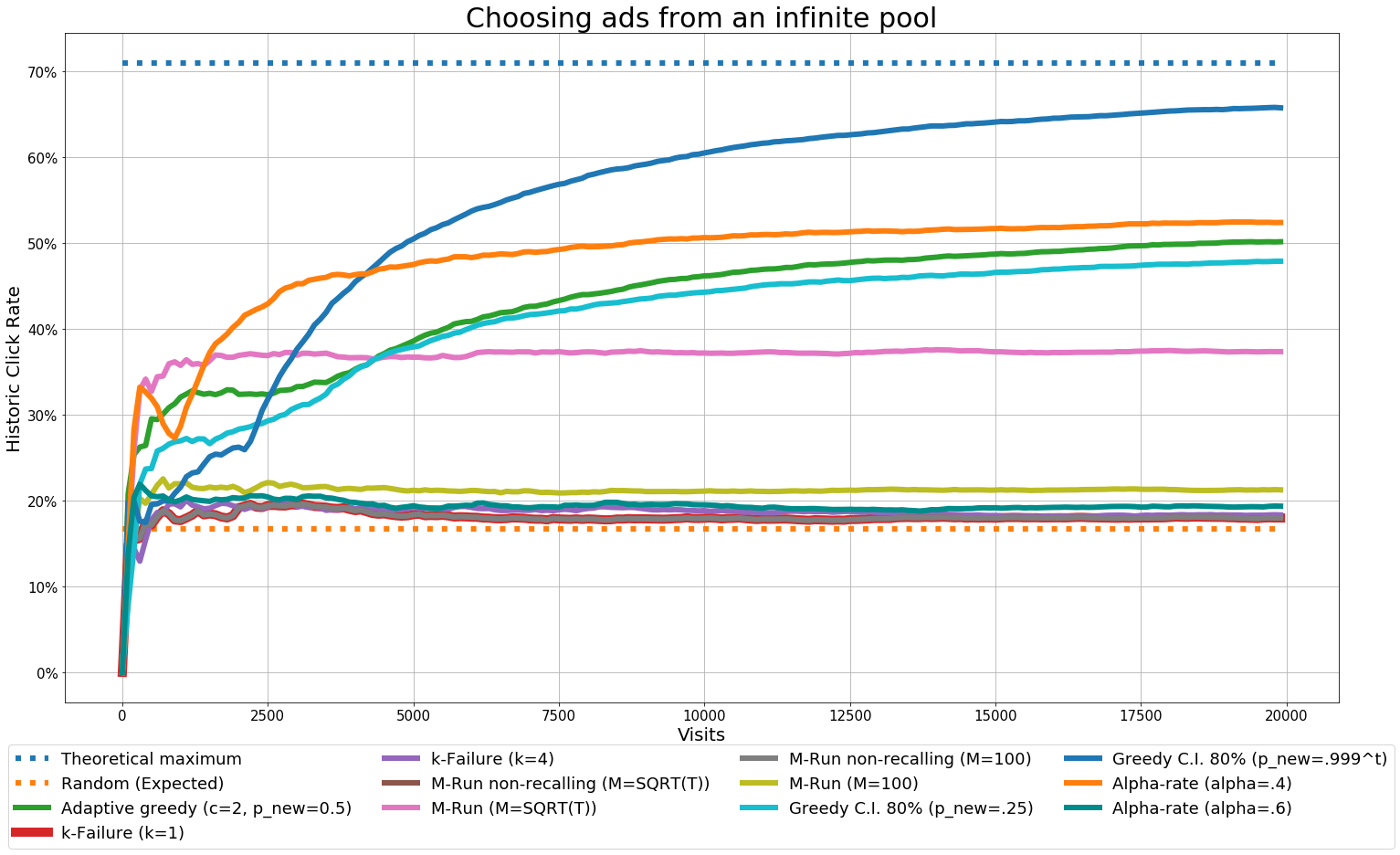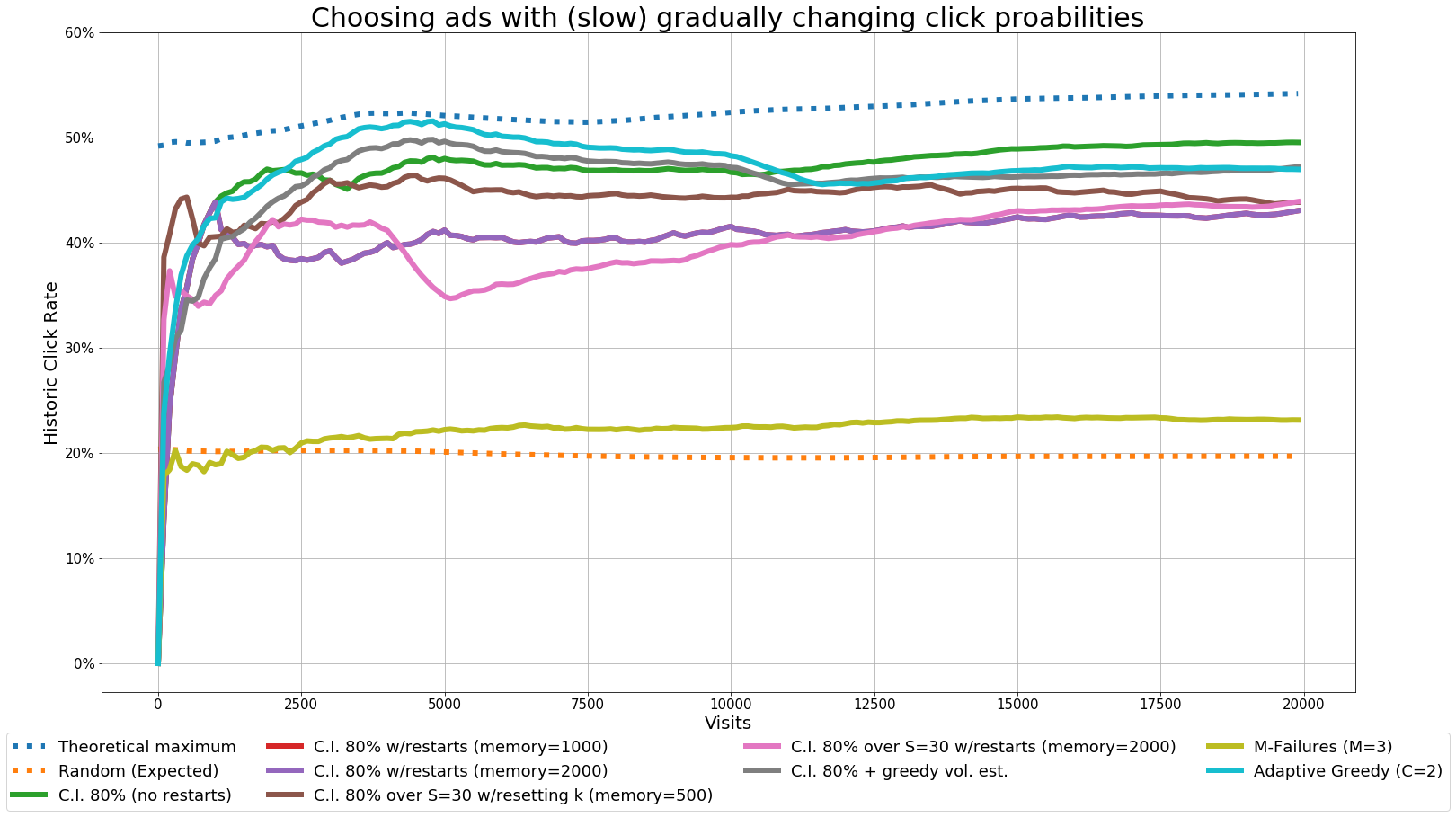Note: if you are visualizing this notebook directly from GitHub, some mathematical symbols might display incorrectly or not display at all. This same notebook can be rendered from nbviewer by following this link.

This project consists of comparing different policies for how to choose an ad to show amongst a large pool of ads in different situations, using only information about their historical clicks and impressions (that is, no features). The objective is, intuitively, to show the ads that would give the most clicks every time.

This is known as the multi-armed bandits problem, and the challenge is that, if an algorithm/policy is constantly showing new ads to see how well they do, it would get a low average click rate, whereas if it always shows the same ads, it might miss the fact that there are other, better ads to show (known as the exploration/exploitation dilemma).

Here I’ll compare different approaches by making simulations under different situations, assuming that each ad has an inherent probability of being clicked when shown (this probability is not known) and changing some of the conditions (e.g. equal vs. different payment per click, few vs. many, permanent vs. expiring, fixed vs. changing probabilities).

## Sections¶

1. Common algorithms

2. Infinite pool of ads (many-armed bandits)

3. Changing click probabilities (restless bandits)

4. Expiring ads (mortal multi-armed bandits)

## 1 Common algorithms¶

The most successful and widely studied families of algorithms for this problem have consistently being those that create upper confidence bounds on the click probability of each ad based on observed clicks and impressions, and those that randomly or greedily alternate steps of exploitation (showing what has historically been the best ad) and exploration (showing a different ad).

In the most general setting of the multi-armed bandits problem, when the rewards of each arm (here: the click probabilities of each ad) can follow arbitrary distributions (e.g. not just click/no-click, but perhaps something more), upper bounds can be calculated using Hoeffdings inequality and decaying the probability of the sum of variables being less than something with time (e.g.$p=t^{-4}$) – giving the following formula at each time step $T$ for each ad $i$ (known as UCB1):

$$Upper\: bound \:\hat{P_i} = \frac{\sum_t Clicks_{i,t}}{\sum_t Displays_{i,t}} + \sqrt{ \frac{2 \times ln \,T}{\sum_t Displays_{i,t}} }$$

However, as in this case the clicks are known to follow a Bernoulli distribution (i.e. we know that they can only be click or no-click, each with a certain probability), it's possible to build better upper confidence bounds using classical statistical approximations for the confidence interval of a rate.

Let $\hat{P_i} = \frac{\sum_t Clicks_{i,t}}{\sum_t Displays_{i,t}}$ and $Z_{inv}(x)$ be the z-score in a normal distribution at which $P(\frac{X-\bar{X}}{\sigma} \ge Z) = x$, then:

$$Confidence \: Interval \: (P_i, \alpha) = \hat{P_i} + Z_{inv}(\alpha) \times \sqrt{\frac{\hat{P_i}\times(1-\hat{P_i})}{\sum_t Displays_{i,t}}}$$
_Note: this is an approximation only, but holds pretty well for large samples and non-extreme rates._

Then, at each opportunity, the ad with the highest upper confidence bound on its estimated click rate is shown.

Under bayesian assumptions, a potential policy to follow is to establish prior distributions for the rates of each ad (such as a beta distribution given by a=clicks,b=impressions-clicks), sample each parameter randomly form their prior distributions, and show the ad with the highest such sampled parameter (known as Thompson sampling), then updating the priors.

In the family of randomized exploration/exploitation algorithms, algorithms known as Epsilon-Greedy take at each step an exploration move (show a random ad) or exploitation move (show the ad with the highest historical click rate) with a certain probability.

Here I'll compare different instantiations (using different parameters) of these algorithms just described: UCB1, Upper Confidence Interval, Thompson Sampling, Epislon-Greedy.

The performance of algorithms for the multi-armed bandits problem can studied and evaluated in terms of their regret - that is, the difference between selecting, at each opportunity, the ad that had the highest click probability vs. the ad that the policy chooses, accummulated in time.

It can be proven mathematically that the regret of UCB algorithms and Thompson sampling can be upper bounded, as T tends to infinite, by $O(K ln T)$ (where K is the number of ads and T is the time), and it can be proved that no algorithm can achieve an asymptotically better bound than this (meaning: the accumulated regret of a good algorithm should only increase logarithmically with time - something that increases linearly (meaning: the ad selection criteria doesn't improve after collecting more clicks and impressions) would be bad).

However, this is only an asymptotic bound and in practice different algorithms with the same asymptotic bound can achieve quantitatively different results at any given non-infinite time.

### 1.1 Classical setting¶

In order to compare different ranking policies, I'll start with a simulation of the most studied formulation of this problem, given as follows:

• At each step or turn, one ad has to be selected to be shown from a pool of n ads.
• Each ad has a given probability of being clicked when shown, which is constant through time.
• Every time an ad is shown, it’s known whether it was clicked or not, and this is the only information given about ads.
• Each click is equally good.

(In the literature, the ads would be known as 'arms' - as in the arms of a one-armed bandit gambling machine - and the clicks received as rewards)

Here I’ll simulate the environment as follows:

• Probabilities of ads being clicked as coming from a beta distribution ($P(Click_{ad}) \sim Beta(2,17)$)
• 100,000 time steps (i.e. 100,000 visits or impressions).
• When appropriate, algorithms start with an assumption (or prior distribution modeled as beta(1,1)) of each ad having a 50% click rate.

Which ad would have gotten a click at each time step will be decided at the beginning of each iteration, thus each algorithm will get the exact same environment (not just the same probabilities) and, should they make the same choices, they will get the exact same number of clicks.

In :
import numpy as np, matplotlib.pyplot as plt
from pylab import rcParams
%matplotlib inline

np.random.seed(159)

# counters keeping track of what each algorithm sees
ctr_eg1=list()

ctr_eg_dec=list()

ctr_ucb1=list()

ctr_ci8=list()

ctr_ci95=list()

ctr_thomp=list()

# initiating the simulation
np.random.seed(123)
for i in range(100000):
if np.random.random()<=.1:
else:

if np.random.random()<=.1*(.999**i):
else:

rate_ci8=clicks_ci8/trials_ci8

rate_ci95=clicks_ci95/trials_ci95

# determining clicks

# collecting CTR every 100 steps
if (i%100)==0:
ctr_thomp.append(np.sum(clicks_thomp)/np.sum(trials_thomp))

# visualizing teh results
rcParams['figure.figsize'] = 25, 15
lwd=5
plt.plot(ctr_ucb1,label='UCB1',linewidth=lwd)
plt.plot(ctr_eg1,label='Epsilon-Greedy (e=0.1)',linewidth=lwd)
plt.plot(ctr_eg_dec,label='Epsilon-Greedy (e=0.1*0.999^t)',linewidth=lwd)
plt.plot(ctr_ci8,label='C.I. 80%',linewidth=lwd)
plt.plot(ctr_ci95,label='C.I. 95%',linewidth=lwd)
plt.plot(ctr_thomp,label='Thompson sampling',linewidth=lwd)
plt.grid()

ax = plt.subplot(111)
box = ax.get_position()
ax.set_position([box.x0, box.y0 + box.height * 0.1,
box.width, box.height * 0.9])
ax.legend(loc='upper center', bbox_to_anchor=(0.5, -0.05),
fancybox=True, ncol=3, prop={'size':20})

plt.tick_params(axis='both', which='major', labelsize=15)
ax.xaxis.set_ticklabels(+[100*i for i in range(0,1000+200,200)])

plt.xlabel('Visits',size=20)
plt.ylabel('Historic Click Rate',size=20)
plt.show()Here the Epsilon-Greedy with decaying explore probability didn't manage to explore enough and got stuck with an inferior ad. Also note that while the UCB, UCB throgh C.I. and Thompson sampling algorithms have the same asymptotic performance, there is a marked difference in their performance at any given non-infinite time.

### 1.2 Different prices and multiple ads shown¶

The same algorithms can be easily extended to the case of multiple ads shown at each opporunity - say, selecting 5 ads to show instead of just 1 - and different rewards per ad (e.g. each ad pays a different ammount per click received, which is independent of its click-through rate), by selecting the ad with the highest expected upper bound of payoff, i.e. $argmax \: Revenue_{i} \times UCB(\hat{P_i})$.

Only in the case of Epislon-Greedy algorithms, there is a bit of performance loss with respect to the UCB classes of algorithms, as they still randomly select ads regardless of their click value.

Here I'll simulate the same situation as before, but this time:

• Five ads are shown at each opportunity, and their click probabilities are independent of each other (perhaps not entirely reflective of internet advertising).
• Each ad has a price paid per click, which is simulated as a random variable from a gamma distribution.
In :
# similar simulation as before

# this time, ads have a price per click
np.random.seed(159)

avg_rev_eg1=list()

avg_rev_eg_dec=list()

avg_rev_ucb1=list()

avg_rev_ci8=list()

avg_rev_ci95=list()

avg_rev_thomp=list()

rev_eg1=0
rev_eg_dec=0
rev_ucb1=0
rev_ci8=0
rev_ci95=0
rev_thomp=0

np.random.seed(123)
for i in range(100000):
if np.random.random()<=.1:
else:

if np.random.random()<=.1*(.999**i):
else:

rate_ci8=clicks_ci8/trials_ci8

rate_ci95=clicks_ci95/trials_ci95

# determining clicks

if (i%100)==0:
avg_rev_eg1.append(rev_eg1/(i+1))
avg_rev_eg_dec.append(rev_eg_dec/(i+1))
avg_rev_ucb1.append(rev_ucb1/(i+1))
avg_rev_ci8.append(rev_ci8/(i+1))
avg_rev_ci95.append(rev_ci95/(i+1))
avg_rev_thomp.append(rev_thomp/(i+1))

rcParams['figure.figsize'] = 25, 15
lwd=5
plt.plot([max_value]*int((i+1)/100),linestyle=':',label='Theoretical maximum',linewidth=lwd)
plt.plot(avg_rev_eg1,label='Epsilon-Greedy (e=0.1*0.999^t)',linewidth=lwd)
plt.plot(avg_rev_eg_dec,label='Epsilon-Greedy (e=0.1)',linewidth=lwd)
plt.plot(avg_rev_ucb1,label='UCB1',linewidth=lwd)
plt.plot(avg_rev_ci8,label='C.I. 80%',linewidth=lwd)
plt.plot(avg_rev_ci95,label='C.I. 95%',linewidth=lwd)
plt.plot(avg_rev_thomp,label='Thompson sampling',linewidth=lwd)
plt.grid()

ax = plt.subplot(111)
box = ax.get_position()
ax.set_position([box.x0, box.y0 + box.height * 0.1,
box.width, box.height * 0.9])
ax.legend(loc='upper center', bbox_to_anchor=(0.5, -0.05),
fancybox=True, ncol=3, prop={'size':20})

plt.tick_params(axis='both', which='major', labelsize=15)
ax.xaxis.set_ticklabels(+[100*i for i in range(0,1000+200,200)])

plt.xlabel('Visits',size=20)
plt.ylabel('Historical Revenue per visit',size=20)
plt.show()As expected, nothing changed much with respect to the previous situation.

## 2. Infinite pool of ads (many-armed bandits)¶

Algorithms based on upper-confidence bounds start by exploring all the different ads, thus if there are an infinite number of ads, or a number of ads larger than the number of times that an ad will be shown, their performance is the same as just selecting an ad at random.

In such settings (known as many-armed bandits), algorithms based on trying one ad at a time and switching to a new ad based on numbers of consecutive failures for a certain number of times can have a provable sub-linear asymptotic bound on their regret, as described in Berry, D. A., Chen, R. W., Zame, A., Heath, D. C., & Shepp, L. A. (1997). Bandit problems with infinitely many arms. The Annals of Statistics, 2103-2116., Herschkorn, S. J., Pekoez, E., & Ross, S. M. (1996). Policies without memory for the infinite-armed Bernoulli bandit under the average-reward criterion. Probability in the Engineering and Informational Sciences, 10, 21-28. and Teytaud, O., Gelly, S., & Sebag, M. (2007). Anytime many-armed bandits. In CAP07., if the number of time steps/iterations/visits is known an the number of failures is established according to it.

While not having a mathematically provable bound on their regret, other families of greedy algorithms can in practice have even better performance than failure-based algorithms, such as those based on dropping an ad if its empirical performance falls below some threshold (alpha-rate), or other greedy algorithms based on exploring/exploiting according to the empirical performance of the best ad – here I’ll also compare the Adaptive Greedy algorithm as described in Chakrabarti, D., Kumar, R., Radlinski, F., & Upfal, E. (2009). Mortal multi-armed bandits. In Advances in neural information processing systems (pp. 273-280)., with the slight modification of, in exploration steps, choosing with a certain probability between taking a new ad or showing an old ad.

Even within the upper confidence bound family of algorithms, variations of them that explore only a subset of the possibilities at a time can achieve a low regret, depending on the underlying distribution of probabilities of each ad (reward distribution of each arm). As well, greedy algorothms based on exploiting old ads or taking new ads can also perform well in practice.

Here I’ll simulate such a situation by having as many ads as time steps to show one of them. This is, in practice, the same as having an infinite pool of ads, if we considering that any ad is the same before being selected to be shown. However, as they will already be instantiated in the simulation, it’s still possible to calculate the regret with respect to the best ad rather than with the maximum probability of the distribution from which the click probabilities of each ad are shown (in a beta distribution, that would be a probability of 1).

This time the simulation is with object-oriented programming, with each algorithm taking a new ad from a pool of ads that shows them in the exact same order for all, and as well, the clicks that each ad would have received determined at the beginning of each iteration and being the same for all ads.

In :
class manyarmed_bandit:
def __init__(self,ordered_clickprob):
self.clicks=np.array([])
self.displays=np.array([])

# for failure-based methods
self.m=10
self.k_failures=1
self.chosen_m=None

# for alpha-rate
self.alpha=0.4

self.greedy_c=2

# parameters
def set_m(self,m):
self.m=m

def set_k_failures(self,k):
self.k_failures=k

def set_alpha(self,alpha):
self.alpha=alpha

def set_greedy_c(self,c):
self.greedy_c=c

# some universal features
def take_new(self):
self.clicks=np.concatenate([self.clicks,])
self.displays=np.concatenate([self.displays,])

def get_ctr(self):
return np.sum(self.clicks)/np.sum(self.displays)

def show_last(self,would_have_clicked):

def show_best_bi_ci(self,would_have_clicked):
if np.sum(self.displays)>0:
ctr=self.clicks/self.displays
else:
self.take_new()
self.show_last(would_have_clicked)

if np.sum(self.clicks)==0:
else:
bestr=np.nanmax(self.clicks/self.displays)
if np.random.random()<self.greedy_c*bestr:
else:
if np.random.random()<p_new:
self.take_new()
self.show_last(would_have_clicked)
else:
# choose an old random ad

# failure-based
if (self.displays[-1]-self.clicks[-1])>=self.k_failures:
self.take_new()
self.show_last(would_have_clicked)

if self.chosen_m is not None:
else:
if (self.clicks[-1]>=self.m) and ((self.displays[-1]-self.clicks[-1])==0):
self.chosen_m=self.clicks.shape-1

if self.chosen_m is not None:
else:
if (self.clicks[-1]>=self.m) and ((self.displays[-1]-self.clicks[-1])==0):
self.chosen_m=np.nanargmax(self.clicks/self.displays)
if self.displays.shape>=self.m:
self.chosen_m=np.nanargmax(self.clicks/self.displays)

# other heuristics
def show_best_by_ci_greedy(self,would_have_clicked,p_new=.5):
if np.random.random()<p_new:
self.take_new()
self.show_last(would_have_clicked)
else:
self.show_best_bi_ci(would_have_clicked)

def show_best_by_alpha_rate(self,would_have_clicked):
if (self.clicks[-1]/self.displays[-1])>=self.alpha:
self.show_last(would_have_clicked)
else:
self.take_new()
self.show_last(would_have_clicked)

In :
# simulation's underlying parameters
generator_par_a=2
generator_par_b=10

# initial arms

bandits_1_failures.take_new()
ctr_1_failures=list()

bandits_4_failures.set_k_failures(4)
bandits_4_failures.take_new()
ctr_4_failures=list()

bandits_m_run_nonrecalling_low_m.set_m(100)
bandits_m_run_nonrecalling_low_m.take_new()
ctr_m_run_nonrecalling_low_m=list()

bandits_m_run_low_m.set_m(100)
bandits_m_run_low_m.take_new()
ctr_m_run_low_m=list()

bandits_m_run_nonrecalling.take_new()
ctr_m_run_nonrecalling=list()

bandits_m_run.take_new()
ctr_m_run=list()

bandits_ci_greedy.take_new()
ctr_ci_greedy=list()

bandits_ci_greedy_decreasing.take_new()
ctr_ci_greedy_decreasing=list()

bandits_alpha_rate.take_new()
ctr_alpha_rate=list()

bandits_alpha_rate2.set_alpha(.65)
bandits_alpha_rate2.take_new()
ctr_alpha_rate2=list()

np.random.seed(777)
# algorithms take ads sequentially, thus at each time step there's only a limited number they can have already taken

######################
bandits_ci_greedy.show_best_by_ci_greedy(would_have_clicked,p_new=.25)
bandits_ci_greedy_decreasing.show_best_by_ci_greedy(would_have_clicked,p_new=1.0*(.999**i))
bandits_alpha_rate.show_best_by_alpha_rate(would_have_clicked)
bandits_alpha_rate2.show_best_by_alpha_rate(would_have_clicked)

######################
if (i%100)==0:
ctr_1_failures.append(bandits_1_failures.get_ctr())
ctr_4_failures.append(bandits_4_failures.get_ctr())
ctr_m_run_nonrecalling_low_m.append(bandits_m_run_nonrecalling_low_m.get_ctr())
ctr_m_run_low_m.append(bandits_m_run_low_m.get_ctr())
ctr_m_run_nonrecalling.append(bandits_m_run_nonrecalling.get_ctr())
ctr_m_run.append(bandits_m_run.get_ctr())
ctr_ci_greedy.append(bandits_ci_greedy.get_ctr())
ctr_ci_greedy_decreasing.append(bandits_ci_greedy_decreasing.get_ctr())
ctr_alpha_rate.append(bandits_alpha_rate.get_ctr())
ctr_alpha_rate2.append(bandits_alpha_rate2.get_ctr())

C:\Users\david\Anaconda3\lib\site-packages\ipykernel\__main__.py:114: RuntimeWarning: invalid value encountered in double_scalars
C:\Users\david\Anaconda3\lib\site-packages\ipykernel\__main__.py:54: RuntimeWarning: invalid value encountered in true_divide

In :
from pylab import rcParams

rcParams['figure.figsize'] = 25, 15

start_from=0
lwd=6

plt.plot(ctr_1_failures[start_from:],label='k-Failure (k=1)',linewidth=10)
plt.plot(ctr_4_failures[start_from:],label='k-Failure (k=4)',linewidth=lwd)
plt.plot(ctr_m_run_nonrecalling_low_m[start_from:],label='M-Run non-recalling (M=SQRT(T))',linewidth=lwd)
plt.plot(ctr_m_run_low_m[start_from:],label='M-Run (M=SQRT(T))',linewidth=lwd)
plt.plot(ctr_m_run_nonrecalling[start_from:],label='M-Run non-recalling (M=100)',linewidth=lwd)
plt.plot(ctr_m_run[start_from:],label='M-Run (M=100)',linewidth=lwd)
plt.plot(ctr_ci_greedy[start_from:],label='Greedy C.I. 80% (p_new=.25)',linewidth=lwd)
plt.plot(ctr_ci_greedy_decreasing[start_from:],label='Greedy C.I. 80% (p_new=.999^t)',linewidth=lwd)
plt.plot(ctr_alpha_rate[start_from:],label='Alpha-rate (alpha=.4)',linewidth=lwd)
plt.plot(ctr_alpha_rate2[start_from:],label='Alpha-rate (alpha=.6)',linewidth=lwd,color='darkcyan')
plt.grid()

ax = plt.subplot(111)
box = ax.get_position()
ax.set_position([box.x0, box.y0 + box.height * 0.1,
box.width, box.height * 0.9])
ax.legend(loc='upper center', bbox_to_anchor=(0.5, -0.05),
fancybox=True, ncol=4, prop={'size':18})

plt.tick_params(axis='both', which='major', labelsize=15)
ax.xaxis.set_ticklabels(+[100*i for i in range(0,225,25)])

plt.xlabel('Visits',size=20)
plt.ylabel('Historic Click Rate',size=20)
plt.title('Choosing ads from an infinite pool',size=30)
plt.show()## 3. Changing click probabilities (restless bandits)¶

All the previous settings assumed that the click probabilities of each ad (the reward distributions of each arm) are static. In practice, however, trends and tastes are constantly changing and the click probabilities of ads can change in the long run.

This problem is known as restless bandits and variations of it under different settings have been studied in other contexts, such as when the clicks are not random but deterministically chosen by an all-powerful adversary in a mini-max context (Auer, P., Cesa-Bianchi, N., Freund, Y., & Schapire, R. E. (2002). The nonstochastic multiarmed bandit problem. SIAM journal on computing, 32(1), 48-77. – even under such conditions it’s still possible to achieve a sub-linear asymptotic regret), when the click probabilities might change abruptly at some point in time (Hartland, C., Gelly, S., Baskiotis, N., Teytaud, O., & Sebag, M. (2006). Multi-armed bandit, dynamic environments and meta-bandits.), or when there is a small number of ads and their probabilities change according to a Wiener process with bound reflections (Slivkins, A., & Upfal, E. (2008). Adapting to a Changing Environment: the Brownian Restless Bandits. In COLT (pp. 343-354). – their methods, however, don’t apply in cases where the number of ads is large).

Here I’ll simulate such a situation by having a score associated to each ad that translates into a click probability by a sigmoid function (defined below), and this score experiencing changes through a random walk, with a different variance for each ad (this is very similar to having log-odds that change through a random walk/Wiener process/Brownian motion, but with a more limited range of click probabilities). Unfortunately, such a setting has not been studied in the literature in the case of a large pool of ads.

If the click probabilities change more slowly than the confidence intervals that UCB algorithms can generate, such algorithms can still achieve near-optimal regret as they are.

In cases of fast-changing probabilities, the same algorithms illustrated at the beginning can also be used if their past memory of clicks and impressions is reset after some iterations (as described too in (Slivkins, A., & Upfal, E. (2008). Adapting to a Changing Environment: the Brownian Restless Bandits. In COLT (pp. 343-354).), or if they explore only a subset of ads at a time and this subset changes in time.

While not possible to estimate the volatility or variance of each ad probability, it’s possible to have an incorrect approximation even if the function that translates a 'score' into click probability is unknown, considering the last estimated click rate of each ad and the last time it was shown, by assuming that the log-odds of the adds follow some random walk with some pre-defined variance (this is, of course, incorrect, but can nevertheless help to determine when it’s time to try an old ad again, in the hopes that its click probability has increased enough).

Coding the algorithms and necessary formulas - note that the objects defined here have methods with the same names as before, but they work differently:

In :
def score_to_prob(score):
return .6/(1+np.exp(-.25*(score-.25)))

def logodds_to_prob(logodds):
return np.exp(logodds)/(1+np.exp(logodds))

def prob_to_logodds(prob):
return np.log(prob/(1-prob))

In :
class restless_bandit_model:

self.acc_clicks=0
self.acc_displays=0

self.k_chosen=set()
self.k=0

self.greedy_c=2

self.last_random=None
self.failures_in_a_row=0
self.m=4

self.est_volatility=.02

def reset(self):
self.acc_clicks+=np.sum(self.clicks)
self.acc_displays+=np.sum(self.displays)

self.k_chosen=set()

self.last_random=None
self.failures_in_a_row=0

best=np.nanargmax(self.clicks/self.displays)

def get_best_r(self):
return np.nanmax(self.clicks/self.displays)

def get_ctr(self):
return (np.sum(self.clicks)+self.acc_clicks)/(np.sum(self.displays)+self.acc_displays)

def choose_k(self,k):
self.k_chosen=set(np.random.choice([i for i in range(self.clicks.shape)],size=k,replace=False))
self.k=k

def show_best_by_ci(self,would_have_clicked,t):
rates=self.clicks/self.displays

def show_best_by_ci_within_k(self,would_have_clicked,t):
rates=self.clicks/self.displays
ci=rates+0.8*np.sqrt(rates*(1-rates)/self.displays)
ci[[i for i in range(self.clicks.shape) if i not in self.k_chosen]]=-1

if np.sum(self.clicks)==0:
else:
bestr=self.get_best_r()
if np.random.random()<self.greedy_c*bestr:
else:

if self.last_random is None:
self.failures_in_a_row=0
if self.failures_in_a_row>=self.m:
self.failures_in_a_row=0
else:

def show_best_by_ci_plus_extravar(self,would_have_clicked,t):
rates=self.clicks/self.displays
est_logodds=np.array([prob_to_logodds(r) for r in rates])
diff_lastshown=t-self.last_shown

extravar=est_logodds+0.8*np.sqrt(diff_lastshown*self.est_volatility)
p_w_extravar=np.array([logodds_to_prob(lpr) for lpr in extravar])

extra_ci=p_w_extravar+0.8*np.sqrt(rates*(1-rates)/self.displays)



### 3.1 Slowly changing probabilities¶

First a simulation having probabilities that change slowly - if they change slowly enough, UCB-based algorithms don't suffer much in their performance.

In :
np.random.seed(6534)

# generating parameters

# generative process
def get_who_would_click():

return would_have_clicked

# models
ctr_ci_w_restarts=list()

ctr_ci_w_restarts2=list()

ctr_ci_no_restart=list()

ci_plus_extravar.est_volatility=0.001
ctr_ci_plus_extravar=list()

ci_over_k.choose_k(30)
ctr_ci_over_k=list()

ci_over_k_w_restarts.choose_k(30)
ctr_ci_over_k_w_restarts=list()

m_failures.m=4
ctr_m_failures=list()

prob_max=list()
prob_avg=list()
ctr_max=list()
ctr_avg=list()

for t in range(20000):
would_have_clicked=get_who_would_click()
#############################

ci_w_restarts.show_best_by_ci(would_have_clicked,t)
ci_w_restarts2.show_best_by_ci(would_have_clicked,t)
ci_no_restart.show_best_by_ci(would_have_clicked,t)
ci_plus_extravar.show_best_by_ci_plus_extravar(would_have_clicked,t)
ci_over_k.show_best_by_ci_within_k(would_have_clicked,t)
ci_over_k_w_restarts.show_best_by_ci_within_k(would_have_clicked,t)

if (t%500)==0 and t>0:
ci_over_k.choose_k(30)

if (t%1000)==0 and t>0:
ci_w_restarts.reset()
ci_over_k_w_restarts.reset()
ci_over_k_w_restarts.choose_k(30)
ci_w_restarts2.reset()

#############################
if (t%100)==0:
ctr_max.append(np.mean(prob_max))
ctr_avg.append(np.mean(prob_avg))

ctr_ci_w_restarts.append(ci_w_restarts.get_ctr())
ctr_ci_w_restarts2.append(ci_w_restarts2.get_ctr())
ctr_ci_no_restart.append(ci_no_restart.get_ctr())
ctr_ci_plus_extravar.append(ci_plus_extravar.get_ctr())
ctr_ci_over_k.append(ci_over_k.get_ctr())
ctr_ci_over_k_w_restarts.append(ci_over_k_w_restarts.get_ctr())
ctr_m_failures.append(m_failures.get_ctr())

C:\Users\david\Anaconda3\lib\site-packages\ipykernel\__main__.py:56: RuntimeWarning: invalid value encountered in true_divide
C:\Users\david\Anaconda3\lib\site-packages\ipykernel\__main__.py:88: RuntimeWarning: invalid value encountered in true_divide
C:\Users\david\Anaconda3\lib\site-packages\ipykernel\__main__.py:61: RuntimeWarning: invalid value encountered in true_divide
C:\Users\david\Anaconda3\lib\site-packages\ipykernel\__main__.py:8: RuntimeWarning: divide by zero encountered in log
C:\Users\david\Anaconda3\lib\site-packages\ipykernel\__main__.py:46: RuntimeWarning: invalid value encountered in true_divide
C:\Users\david\Anaconda3\lib\site-packages\ipykernel\__main__.py:42: RuntimeWarning: invalid value encountered in true_divide
C:\Users\david\Anaconda3\lib\site-packages\ipykernel\__main__.py:8: RuntimeWarning: divide by zero encountered in double_scalars
C:\Users\david\Anaconda3\lib\site-packages\ipykernel\__main__.py:5: RuntimeWarning: invalid value encountered in double_scalars

In :
rcParams['figure.figsize'] = 25, 15

start_from=0
lwd=6
plt.plot(ctr_max[start_from:],label='Theoretical maximum',linestyle=':',linewidth=lwd)
plt.plot(ctr_avg[start_from:],label='Random (Expected)',linestyle=':',linewidth=lwd)

plt.plot(ctr_ci_no_restart[start_from:],label='C.I. 80% (no restarts)',linewidth=lwd)
plt.plot(ctr_ci_w_restarts[start_from:],label='C.I. 80% w/restarts (memory=1000)',linewidth=lwd)
plt.plot(ctr_ci_w_restarts2[start_from:],label='C.I. 80% w/restarts (memory=2000)',linewidth=lwd)
plt.plot(ctr_ci_over_k[start_from:],label='C.I. 80% over S=30 w/resetting k (memory=500)',linewidth=lwd)
plt.plot(ctr_ci_over_k_w_restarts[start_from:],label='C.I. 80% over S=30 w/restarts (memory=2000)',linewidth=lwd)
plt.plot(ctr_ci_plus_extravar[start_from:],label='C.I. 80% + greedy vol. est.',linewidth=lwd)
plt.plot(ctr_m_failures[start_from:],label='M-Failures (M=3)',linewidth=lwd)
plt.grid()

ax = plt.subplot(111)
box = ax.get_position()
ax.set_position([box.x0, box.y0 + box.height * 0.1,
box.width, box.height * 0.9])
ax.legend(loc='upper center', bbox_to_anchor=(0.5, -0.05),
fancybox=True, ncol=4, prop={'size':18})

plt.tick_params(axis='both', which='major', labelsize=15)
ax.xaxis.set_ticklabels(+[100*i for i in range(0,225,25)])

plt.xlabel('Visits',size=20)
plt.ylabel('Historic Click Rate',size=20)
plt.show()### 3.2 Not-so-slowly changing probabilities¶

If, however, the click probabilities change faster, then other greedy algorithms can outperform simple upper confidence bounds that assume probabilites to be static:

In :
np.random.seed(8478)

# generating parameters

# models
ctr_ci_w_restarts=list()

ctr_ci_w_restarts2=list()

ctr_ci_no_restart=list()

ci_plus_extravar.est_volatility=0.0005
ctr_ci_plus_extravar=list()

ci_over_k.choose_k(30)
ctr_ci_over_k=list()

ci_over_k_w_restarts.choose_k(30)
ctr_ci_over_k_w_restarts=list()

m_failures.m=4
ctr_m_failures=list()

prob_max=list()
prob_avg=list()
ctr_max=list()
ctr_avg=list()

for t in range(20000):
would_have_clicked=get_who_would_click()
#############################

ci_w_restarts.show_best_by_ci(would_have_clicked,t)
ci_w_restarts2.show_best_by_ci(would_have_clicked,t)
ci_no_restart.show_best_by_ci(would_have_clicked,t)
ci_plus_extravar.show_best_by_ci_plus_extravar(would_have_clicked,t)
ci_over_k.show_best_by_ci_within_k(would_have_clicked,t)
ci_over_k_w_restarts.show_best_by_ci_within_k(would_have_clicked,t)

if (t%500)==0 and t>0:
ci_over_k.choose_k(30)

if (t%1000)==0 and t>0:
ci_w_restarts.reset()
ci_over_k_w_restarts.reset()
ci_over_k_w_restarts.choose_k(30)
ci_w_restarts2.reset()

#############################
if (t%100)==0:
ctr_max.append(np.mean(prob_max))
ctr_avg.append(np.mean(prob_avg))

ctr_ci_w_restarts.append(ci_w_restarts.get_ctr())
ctr_ci_w_restarts2.append(ci_w_restarts2.get_ctr())
ctr_ci_no_restart.append(ci_no_restart.get_ctr())
ctr_ci_plus_extravar.append(ci_plus_extravar.get_ctr())
ctr_ci_over_k.append(ci_over_k.get_ctr())
ctr_ci_over_k_w_restarts.append(ci_over_k_w_restarts.get_ctr())
ctr_m_failures.append(m_failures.get_ctr())

from pylab import rcParams

rcParams['figure.figsize'] = 25, 15

start_from=0
lwd=6
plt.plot(ctr_max[start_from:],label='Theoretical maximum',linestyle=':',linewidth=lwd)
plt.plot(ctr_avg[start_from:],label='Random (Expected)',linestyle=':',linewidth=lwd)

plt.plot(ctr_ci_no_restart[start_from:],label='C.I. 80% (no restarts)',linewidth=lwd)
plt.plot(ctr_ci_w_restarts[start_from:],label='C.I. 80% w/restarts (memory=1000)',linewidth=lwd)
plt.plot(ctr_ci_w_restarts2[start_from:],label='C.I. 80% w/restarts (memory=2000)',linewidth=lwd)
plt.plot(ctr_ci_over_k[start_from:],label='C.I. 80% over S=30 w/resetting k (memory=500)',linewidth=lwd)
plt.plot(ctr_ci_over_k_w_restarts[start_from:],label='C.I. 80% over S=30 w/restarts (memory=2000)',linewidth=lwd)
plt.plot(ctr_ci_plus_extravar[start_from:],label='C.I. 80% + greedy vol. est.',linewidth=lwd)
plt.plot(ctr_m_failures[start_from:],label='M-Failures (M=3)',linewidth=lwd)
plt.grid()

ax = plt.subplot(111)
box = ax.get_position()
ax.set_position([box.x0, box.y0 + box.height * 0.1,
box.width, box.height * 0.9])
ax.legend(loc='upper center', bbox_to_anchor=(0.5, -0.05),
fancybox=True, ncol=4, prop={'size':18})

plt.tick_params(axis='both', which='major', labelsize=15)
ax.xaxis.set_ticklabels(+[100*i for i in range(0,225,25)])

plt.xlabel('Visits',size=20)
plt.ylabel('Historic Click Rate',size=20)

C:\Users\david\Anaconda3\lib\site-packages\ipykernel\__main__.py:56: RuntimeWarning: invalid value encountered in true_divide